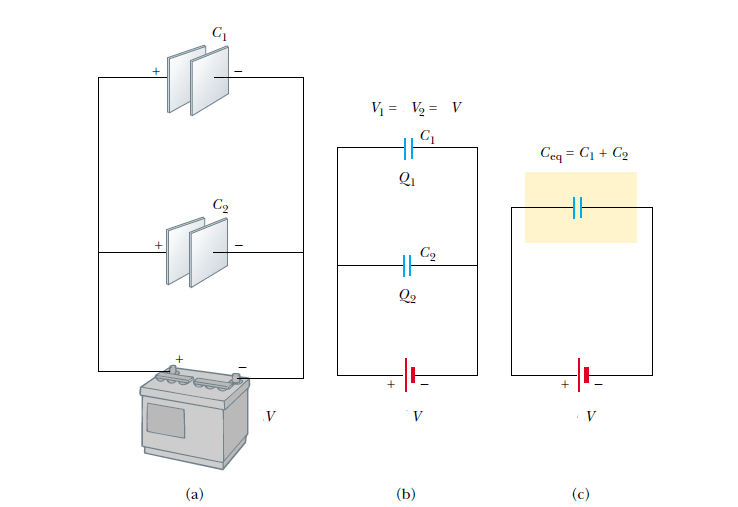# Parallel Combination of capacitors

## Parallel Combination of capacitors

Two capacitors connected as shown in Figure 1a are known as a parallel combination of capacitors. Figure 1b shows a circuit diagram for this combination of capacitors.

The left plates of the capacitors are connected by a conducting wire to the positive terminal of the battery and are therefore both at the same electric potential as the positive terminal. Likewise, the right plates are connected to the negative terminal and are therefore both at the same potential as the negative terminal.figure 1: (a) A parallel combination of two capacitors in an electric circuitin which the potential difference across the battery terminals is V. (b) The circuit diagramfor the parallel combination. (c) The equivalent capacitance is Ceq = C1 + C2.

## Characteristics of Parallel Combination of capacitors

• In parallel combination of capacitors the individual potential differences across capacitors connected in parallel are the same and are equal to the potential difference applied across the combination.
• The total charge on capacitors connected in parallel is the sum of the charges on the individual capacitors.
• The equivalent capacitance of a parallel combination of capacitors is the algebraic sum of the individual capacitances and is greater than any of the individual capacitances. Ceq = C1 + C2 + C3 +… (parallel combination) . ( see derivation of equivalent capacitance of a parallel combination of capacitors)
• When two or more capacitors are connected in parallel, the potential difference across each is the same. The charge on each capacitor is proportional to its capacitance; hence, the capacitances can be added directly to give the equivalent capacitance of the parallel combination. The equivalent capacitance is always larger than the individual capacitances.